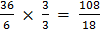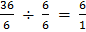### Sample Problem

Complete the ratio table.

 36 6 a 18 6 b 12 2

a:

b:

#### Solution

Equivalent fractions represent equivalent ratios.

Then, start with 36:6. Write the ratio as a fraction. Multiply or divide the numerator and denominator by the same number to find an equivalent ratio., so a = 108, so b = 1